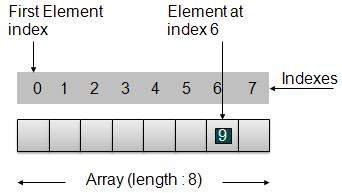# DSA using Java - Arrays

## Array Basics

Array is a container which can hold fix number of items and these items should be of same type. Most of the datastructure make use of array to implement their algorithms. Following are important terms to understand the concepts of Array

• Element − Each item stored in an array is called an element.

• Index − Each location of an element in an array has a numerical index which is used to identify the element.

## Array RepresentationAs per above shown illustration, following are the important points to be considered.

• Index starts with 0.

• Array length is 8 which means it can store 8 elements.

• Each element can be accessed via its index. For example, we can fetch element at index 6 as 9.

## Basic Operations

Following are the basic operations supported by an array.

• Insertion − add an element at given index.

• Deletion − delete an element at given index.

• Search − search an element using given index or by value.

• Update − update an element at given index.

In java, when an array is initialized with size, then it assigns defaults values to its elements in following order.

Data Type Default Value
byte0
short0
int0
long0L
float0.0f
double0.0d
char'\u0000'
booleanfalse
Objectnull

## Demo

```package com.tutorialspoint.array;

public class ArrayDemo {
public static void main(String[] args){

// Declare an array
int intArray[];

// Initialize an array of 8 int
// set aside memory of 8 int
intArray = new int;

System.out.println("Array before adding data.");

// Display elements of an array.
display(intArray);

// Operation : Insertion
// Add elements in the array
for(int i = 0; i< intArray.length; i++)
{
// place value of i at index i.
System.out.println("Adding "+i+" at index "+i);
intArray[i] = i;
}
System.out.println();

System.out.println("Array after adding data.");
display(intArray);

// Operation : Insertion
// Element at any location can be updated directly
int index = 5;
intArray[index] = 10;

System.out.println("Array after updating element at index " + index);
display(intArray);

// Operation : Search using index
// Search an element using index.
System.out.println("Data at index " + index + ": "+ intArray[index]);

// Operation : Search using value
// Search an element using value.
int value = 4;
for(int i = 0; i< intArray.length; i++)
{
if(intArray[i] == value ){
System.out.println(value + " Found at index "+i);
break;
}
}
System.out.println("Data at index " + index + ": "+ intArray[index]);
}

private static void display(int[] intArray){
System.out.print("Array : [");
for(int i = 0; i< intArray.length; i++)
{
// display value of element at index i.
System.out.print(" "+intArray[i]);
}
System.out.println(" ]");
System.out.println();
}
}
```

If we compile and run the above program then it would produce following result −

```Array before adding data.
Array : [ 0 0 0 0 0 0 0 0 ]

Adding 0 at index 0
Adding 1 at index 1
Adding 2 at index 2
Adding 3 at index 3
Adding 4 at index 4
Adding 5 at index 5
Adding 6 at index 6
Adding 7 at index 7

Array after adding data.
Array : [ 0 1 2 3 4 5 6 7 ]

Array after updating element at index 5
Array : [ 0 1 2 3 4 10 6 7 ]

Data at index 5: 10
4 Found at index: 4
```